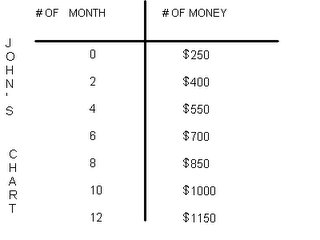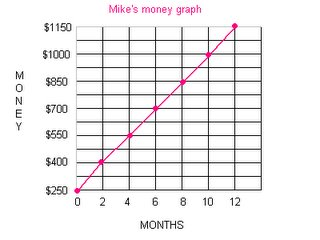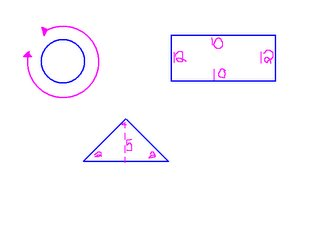## Tuesday, June 06, 2006

### Kim's Growing Post for June 6th

Question 1: Formulas. We've learned how to write algebraic equations in the form of mx + b = y
Convert these two sentences into algebraic using the above formula.

m: change or coefficient
b: constant or start
y: solution

Sentence 1: Scott is 2 years older than Donald who turns 12 on June 21st 2006. Write this sentence in the format of mx + b = y, then convert it into an expression which allows us to figure out the age of Scott. Scott is 13 years old and Donald will be 12 years old.

2 + b = y
2 + 11 = y
2 + 11 = 13
Sentence 2: Elizabeth has \$200 in her savings account. She makes \$40 every two weeks babysitting for her next door neighbours. If Elizabeth saves all of her money solve for how much money she will have in one year? Elizabeth will have a total of \$1160 in one year.
\$40n + \$200 = y
\$40(24) + \$200 = \$1160
Question 2: Creation. You need to create two questions for your classmates that cover different concepts (ex: T-charts, patterns, equations, graphs etc.) that you have learned in this unit on Algebra. You then need to show how to solve the questions. Your mark will be based upon the lvl of question's difficulty, and the effort put into your answer.
Bobby has \$500 dollars in his bank account and he earns \$50 every month. His friend John has \$250 in his bank account and he earns \$75 every month. How much will Bobby and John have in their bank account in one year and who will have the most amount of money? Bobby will have a total of \$1100 and John will have a total of \$1150 and John will have \$50 more than Bobby.
\$50x + \$500 = y
\$50(12) + \$500 = \$1100
\$75x + \$250 = y
\$75(12) + \$250 = \$1150One day, Sandy and Lina decided to save money and buy a walkman for \$150 and share it with each other since they were sisters. Sandy had \$60 in her bank account and she would add \$10 everyday for 10 days while Lina had \$120 in her bank account but she would spend \$10 everyday for herself for 10 days. On the 10th day, will Lina and Sandy have enough money to buy the \$150 walkman and have leftover money? Or will they have to save more to buy a walkman? Sandy and Lina will have enough money in total to buy the \$150 walkman and have \$40 leftover.

Sandy: 10x + 60 = y

10(11) + 60 = 170

Lina: -10x + 120 = y

-10(10) + 120 = 20

Question 3: Reflection. You need to look back at the chart that we filled out during the first day of the unit. This is the chart where you coloured a topic red, yellow or green. You now need to pick one concept that you coloured yellow or red and reflect in words what new skill/idea that you have learned on this topic.

Can I use my words to create an algebraic formula?

I coloured this in red because I didn't know how to create a algebraic formula. So if I didn't know how to make one, I would probably not be able to make one from a T-Chart or use an algebraic formula to solve for an unknown. It was easier to make a formula when Mr.Reece had taught us how and it was helping me solve an unknown and make a formula by using a T-Chart.

Question 4: Preparing for the final exam. You need to think about the year that has past in mathematics and decide which topic is your weakest, and what you need to learn duriong class review in order to prepare yourself for the final exam. It is not enough to say fractions, instead pick your weakest area of fractions, say the subtraction or fractions, and give an example of what you don't understand.

My weakest topic is probably algebra. In algebra, there are always some questions that I can't figure out how to solve the unknown. But as Mr.Reece is teaching us, I am beginning to find it more easy little at a time and will be able to solve it. I also know that when the higher the grade i'm in, the harder the level of mathematics are and the harder the questions in algebra. (eaxample: -50x + 2o6 = y)

KIM-THANH VO ROOM 7-43

## Monday, June 05, 2006

### My Growing Post

Question 1: Formulas. We've learned how to write algebraic equations in the form of mx+b=y. Convert these two sentences into algebraic equations using the above formula.

m - coefficient (change)

x - variable

b - constant (start)

Sentence 1: Scott is 2 years older than Donald who turns 12 on June 21st 2006. Write this sentence in the format mx+b=y, then convert it into an expression which allows us to figure out the age of Scott.

2+d=s
2+11=13

Sentence 2: Elizabeth has \$200 in her savings account. She makes \$40 every two weeks babysitting for her next door neighbours. If Elizabeth saves all of her money solve for how much money she will have in one year.

40+200e=
40 +200(26)=5240

Question 2: Creation. You need to create two questions for your classmates that cover different concepts (ex: T-Charts, patterns, equations, graphs etc.) that you have learned in this unit on Algebra. You then need to show how to solve the questions. Your mark will be based upon the level of questions difficulty, and the effort put into your answer.

There are 500 chocolate muffins for sale at the bake sale. If there were 50 customers and the each got the same amount how many muffins did each customer get?

500/50=n
n=10

There are 12 dentists and 48 toothbrushes. How many toothbrushes does each dentist get?

48/12=n
n=4

Question 3: Reflection. You need to look back at the chart that we filled out during the first day of the unit. This is the chart where you coloured a topic red. yellow or green. You now need to pick one concept that you coloured yellow or red and reflect in words what new skill/idea you have learned on this topic.

Can I create a T-Chart for my pattern?
This topic I coloured red because I did not get how to do that.
Now I can easily do that because it is really easy now that I know what I am doing. I learned that you can solve a pattern by using a T-Chart.

Question 4: Preparing for the final exam. You need to think about the year that has past in mathematics and decide which topic is your weakest, and what you need to learn during class reveiw in order to prepare yourself for the final exam. It is not enough to say fractions, instead pick your weakest area of fractions, say the subtraction of fractions, and give an example of what you don't understand.

My weakest topic is fractions. Inside fractions I have trouble multipling fractions. I have always had trouble with fractions.

Example: 1/4 x 3/4

Kendra 7-43

## Sunday, June 04, 2006

### My Growing post

1. m - coefficient ( change )
x - variable
b- constant ( start )
y - solution ( answer )

S1. 2+N=s
2+11=13

S2. 40+200N=
40+200(26)=5240
Elizabeth will have \$5240 in her acount at the end of the year.

2. There are 156 cookies in a jar and Heather ate 4 a day. How many cookies would be left in the jar at the end of the month which has 30 days?
4/156C=
C = 39

There are 20 Police Officers and 5 dozen doughnuts. How many doughnuts were left for the next day?
20/5P=
P = 4

3. Can I use my words to create an Algebraic Formula?
I coloured this topic red because at the begining of the year I didn't understand how to do it. Now I can make an Algebraic Formula with words and it will make sence.

4. My weakest topic in math is doing Area and Circumference of a Circle, Triangle and Rectangle. I have trouble getting the answers for them.
Example:Thank-you Mr.Reece

### Janna's Growing Post for June 6th

Question 1: Formulas. We’ve learned how to write algebraic equations in the form of
mx + b = y. Convert these two sentences into algebraic equations using the above formula.

m - coefficient ( change )
x - variable
b - constant ( start )
y - solution

Sentence 1. Scott is 2 years older than Donald who turns 12 on June 21st 2006. Write this sentence in the format of mx + b = y, then convert it into an expression which allows us to figure out the age of Scott.
Scott = Donald + 2
Scott = 11 + 2
Scott = 13

N + 2 = 13 N = 11 which is Donalds age since he has not yet turned 12.

Sentence 2. Elizabeth has \$200 in her savings account. She makes \$40 every two weeks babysitting for her next door neighbours. If Elizabeth saves all of her money solve for how much money she will have in one year?

40p + 200 = 1240
- 200 - 200
____ ____
0 1040

40p = 1040

Question 2: Creation. You need to create two questions for your classmates that cover different concepts (ex: T-Charts, patterns, equations, graphs etc.) that you have learned in this unit on Algebra. You then need to show how to solve the questions. Your mark will be based upon the level of question’s difficulty, and the effort put into your answer.

Question 3: Reflection. You need to look back at the chart that we filled out during the first day of the unit. This is the chart where you coloured a topic red, yellow or green. You now need to pick one concept that you coloured yellow or red and reflect in words what new skill/idea that you have learned on this topic.

Question 4: Preparing for the final exam. You need to think about the year that has past in mathematics and decide which topic is your weakest, and what you need to learn during class review in order to prepare yourself for the final exam. It is not enough to say fractions, instead pick your weakest area of fractions, say the subtraction of fractions, and give an example of what you don’t understand.